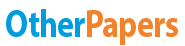# Centripetal Force

Essay by   •  February 12, 2012  •  Research Paper  •  957 Words (4 Pages)  •  1,916 Views

## Essay Preview: Centripetal Force

Report this essay
Page 1 of 4

Centripetal Force

Alexis and Austin

Period 6

January 19, 2012

Objectives:

1. To find out how centripetal force is related to velocity, mass, and radius of the circular path.

2. To determine the m/r ratio for an object undergoing circular motion

Data:

Suspended Mass (kg) Suspended Weight (N) Time for 30 Rev. (s) Time for One Rev. (s) Circumference (m) Speed (m/s)

.0553 .542 29.1 .97 6.28 6.47

.10578 1.037 22.05 .735 6.28 8.54

.15535 1.522 17.94 .598 6.28 10.5

.20526 2.012 15.42 .514 6.28 12.22

.25524 2.501 13.69 .466 6.28 13.49

Measured Mass of Stopper: _______0.01377______ kg

Measured Radius of Circular Path: 1.00 m

Data Treatment:

Weight = (9.8m/s^2) x (Mass)

Weight = (9,8m/s^2) x (.0553kg) = .542N

Time for One Rev = (Time for 30 rev)/ (30 rev)

Time for One Rev = (29.1s)/(30 rev) = .97s

Circumference = 2 ᴨ x (1m) = 6.28m

Speed = distance traveled / time traveled

Speed = 6.28m/.97s = 6.47m/s

Centripetal Force = (mass x velocity^2 )/ radius

Centripetal Force = .01377kg x velocity^2 /1m = .01377kg/m x velocity^2

Centripetal Force = (mass x velocity^2 )/ radius

Centripetal Force = (5kg x( 5m/s)^2)/5m = 25 N

Centripetal Force = (mass x velocity^2)/ radius

25N = (10kg x velocity^2)/5m

125N*m = 10 kg x velocity^2

12.5m^2/s^2 = velocity^2

3.5 m/s = velocity

25N = (5kg x velocity^2)/10m

250N*m = 5kg x velocity^2

50 m^2/s^2 = velocity^2

7.1m.s = velocity

Percentage of Error = |accepted value - experimental value| / accepted value x 100%

Percentage of Error = |(.01377kg/m - .01370kg/m)| / (.01377kg/m) x 100% = .508% Error

Discussion:

1.Plot a graph of centripetal force (suspended weight) versus speed. Describe the resulting curve. What function of speed appears to vary directly with force?

As the velocity increases on the graph, the centripetal force increases. Since one increases as the other increases, it is a direct relationship; therefore, velocity and centripetal force have a direct relationship.

2.Plot the graph which you think will give a linear relationship (force versus some function of speed). According to this straight line, what function of speed varies directly with force (F  ?) Does this support the form of the equation for centripetal force given in the text?

The square of velocity varies directly with force. This supports the form of the equation for centripetal force given in the text because there is a square of velocity in the equation. The square of velocity is also on the top which means that is directly proportional to the

...

...